# 何为C++对象模型？

C++对象模型可以概括为以下2部分：

1. 1.        语言中直接支持面向对象程序设计的部分

2. 2.        对于各种支持的底层实现机制

c语言中，数据处理数据的操作（函数）是分开来声明的，也就是说，语言本身并没有支持数据和函数之间的关联性。在c++中，通过抽象数据类型（abstract data typeADT），在类中定义数据和函数，来实现数据和函数直接的绑定。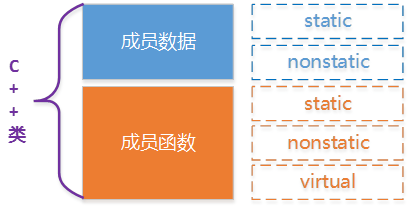Base类定义： #pragma once#includeusing namespace std;class Base{public:    Base(int);    virtual ~Base(void);     int getIBase() const;    static int instanceCount();    virtual void print() const; protected:     int iBase;    static int count;};

Base类在机器中我们如何构建出各种成员数据和成员函数的呢？

# 基本C++对象模型

## 简单对象模型（a simple object model）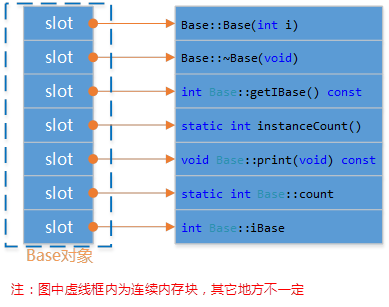## 表格对象模型（a table-driven object model）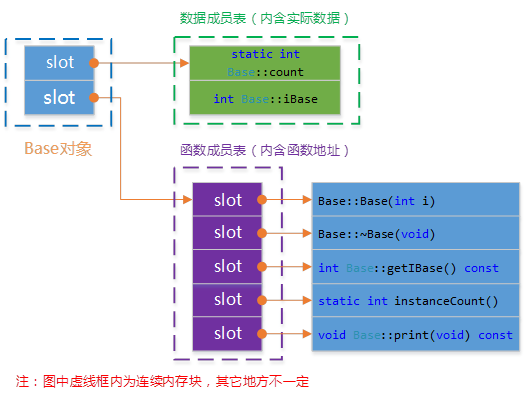## C++对象模型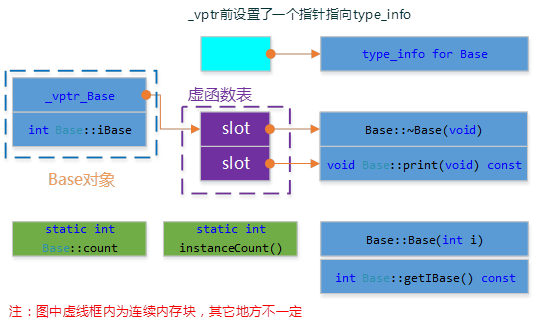1. 1.      每一个class产生一堆指向虚函数的指针，放在表格之中。这个表格称之为虚函数表（virtual tablevtbl）。

2. 2.      每一个对象被添加了一个指针，指向相关的虚函数表vtbl。通常这个指针被称为vptrvptr的设定（setting）和重置（resetting）都由每一个class构造函数析构函数拷贝赋值运算符自动完成。

## 模型验证测试

 模型验证测试： void test_base_model(){    Base b1(1000);    cout << "对象b1的起始内存地址：" << &b1 << endl;    cout << "type_info信息：" << ((int*)*(int*)(&b1) - 1) << endl;    RTTICompleteObjectLocator str=        *((RTTICompleteObjectLocator*)*((int*)*(int*)(&b1) - 1));    //abstract class name from RTTI    string classname(str.pTypeDescriptor->name);    classname = classname.substr(4,classname.find("@@")-4);    cout << classname <

l  虚函数表的中第1个函数地址是虚析构函数地址；

l  虚函数表的中第2个函数地址是虚函数print()的地址，通过函数指针可以调用，进行验证；

l  推测数据成员iBase的地址，为虚函数表的地址 + 1((int*)(&b1) +1)

l  静态数据成员和静态函数所在内存地址，与对象数据成员和函数成员位段不一样；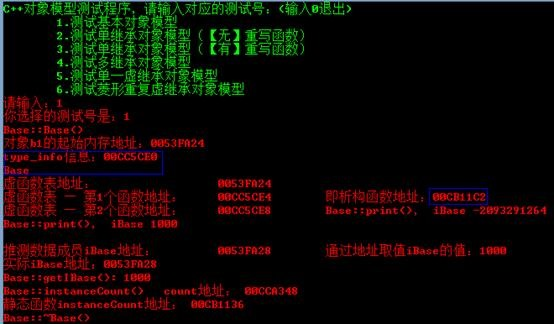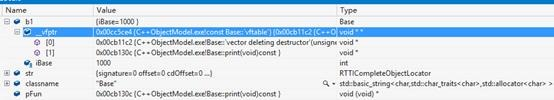# C++对象模型中加入单继承

## 无重写的单继承

 Derived类： #pragma once#include "base.h" class Derived :    public Base{public:    Derived(int);    virtual ~Derived(void);    virtual void derived_print(void); protected:    int iDerived;};

BaseDerived的类图如下所示：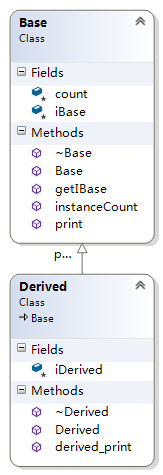Base的模型跟上面的一样，不受继承的影响。Derived不是虚继承，所以是扩充已存在的虚函数表，所以结构如下图所示：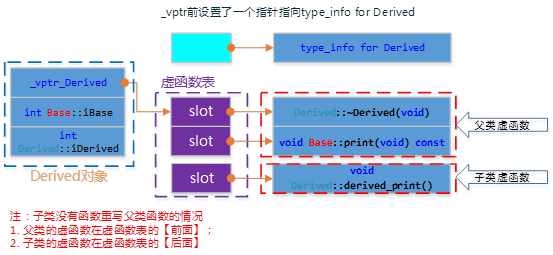测试代码： void test_single_inherit_norewrite(){    Derived d(9999);    cout << "对象d的起始内存地址：" << &d << endl;    cout << "type_info信息：" << ((int*)*(int*)(&d) - 1) << endl;    RTTICompleteObjectLocator str=        *((RTTICompleteObjectLocator*)*((int*)*(int*)(&d) - 1));    //abstract class name from RTTI    string classname(str.pTypeDescriptor->name);    classname = classname.substr(4,classname.find("@@")-4);    cout << classname <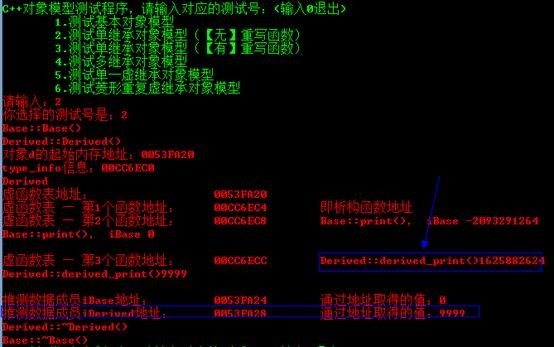## 有重写的单继承

 Derived_Overwrite类： #pragma once#include "base.h"class Derived_Overrite :    public Base{public:    Derived_Overrite(int);    virtual ~Derived_Overrite(void);    virtual void print(void) const; protected:    int iDerived;};

BaseDerived_Overwrite的类图如下所示：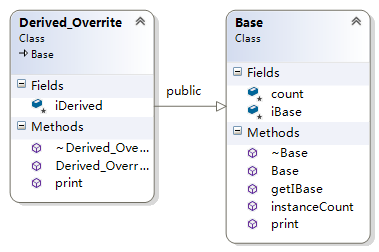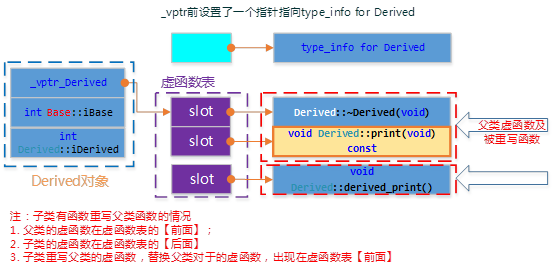测试代码： void test_single_inherit_rewrite(){    Derived_Overrite d(111111);    cout << "对象d的起始内存地址：\t\t" << &d << endl;    cout << "虚函数表地址：\t\t\t" << (int*)(&d) << endl;    cout << "虚函数表 — 第1个函数地址：\t" << (int*)*(int*)(&d) << "\t即析构函数地址" << endl;    cout << "虚函数表 — 第2个函数地址：\t" << ((int*)*(int*)(&d) + 1) << "\t";    typedef void(*Fun)(void);    Fun pFun = (Fun)*(((int*)*(int*)(&d)) + 1);    pFun();    d.print();    cout << endl;     cout << "虚函数表 — 第3个函数地址：\t" << *((int*)*(int*)(&d) + 2) << "【结束】\t";    cout << endl;     cout << "推测数据成员iBase地址：\t\t" << ((int*)(&d) +1) << "\t通过地址取得的值：" << *((int*)(&d) +1) << endl;    cout << "推测数据成员iDerived地址：\t" << ((int*)(&d) +2) << "\t通过地址取得的值：" << *((int*)(&d) +2) << endl;}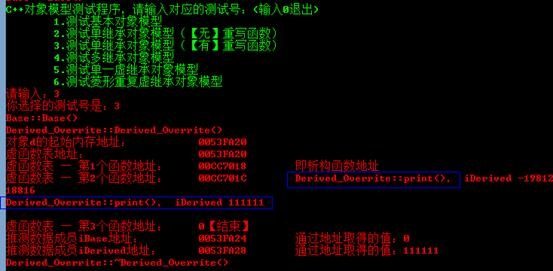# C++对象模型中加入多继承

1. 1)        每个基类都有自己的虚表。

2. 2)        子类的成员函数被放到了第一个基类的表中。

3. 3)        内存布局中，其父类布局依次按声明顺序排列。

4. 4)        每个基类的虚表中的print()函数都被overwrite成了子类的print ()。这样做就是为了解决不同的基类类型的指针指向同一个子类实例，而能够调用到实际的函数。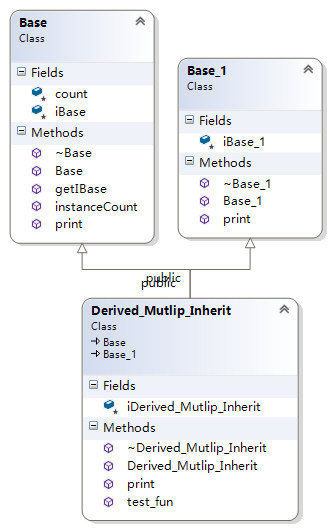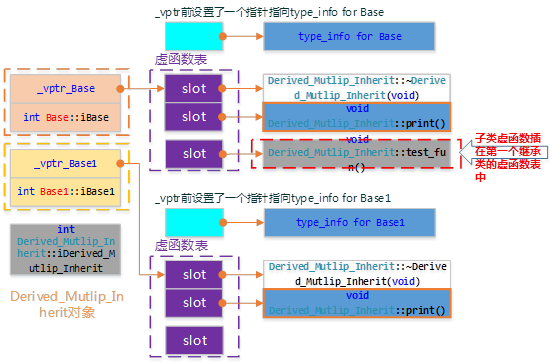测试代码： void test_multip_inherit(){    Derived_Mutlip_Inherit dmi(3333);    cout << "对象dmi的起始内存地址：\t\t" << &dmi << endl;    cout << "虚函数表_vptr_Base地址：\t" << (int*)(&dmi) << endl;    cout << "_vptr_Base — 第1个函数地址：\t" << (int*)*(int*)(&dmi) << "\t即析构函数地址" << endl;    cout << "_vptr_Base — 第2个函数地址：\t" << ((int*)*(int*)(&dmi) + 1) << "\t";    typedef void(*Fun)(void);    Fun pFun = (Fun)*(((int*)*(int*)(&dmi)) + 1);    pFun();    cout << endl;    cout << "_vptr_Base — 第3个函数地址：\t" << ((int*)*(int*)(&dmi) + 2) << "\t";    pFun = (Fun)*(((int*)*(int*)(&dmi)) + 2);    pFun();    cout << endl;    cout << "_vptr_Base — 第4个函数地址：\t" << *((int*)*(int*)(&dmi) + 3) << "【结束】\t";    cout << endl;    cout << "推测数据成员iBase地址：\t\t" << ((int*)(&dmi) +1) << "\t通过地址取得的值：" << *((int*)(&dmi) +1) << endl;      SetConsoleTextAttribute(GetStdHandle(STD_OUTPUT_HANDLE), FOREGROUND_INTENSITY | FOREGROUND_GREEN);     cout << "++++++++++++++++++++++++++++++++++++++++++++++++++++++++++++++" << endl;    SetConsoleTextAttribute(GetStdHandle(STD_OUTPUT_HANDLE), FOREGROUND_INTENSITY | FOREGROUND_RED);     cout << "虚函数表_vptr_Base1地址：\t" << ((int*)(&dmi) +2) << endl;    cout << "_vptr_Base1 — 第1个函数地址：\t" << (int*)*((int*)(&dmi) +2) << "\t即析构函数地址" << endl;    cout << "_vptr_Base1 — 第2个函数地址：\t" << ((int*)*((int*)(&dmi) +2) + 1) << "\t";    typedef void(*Fun)(void);    pFun = (Fun)*((int*)*((int*)(&dmi) +2) + 1);    pFun();    cout << endl;    cout << "_vptr_Base1 — 第3个函数地址：\t" << *((int*)*(int*)((int*)(&dmi) +2) + 2) << "【结束】\t";    cout << endl;       cout << "推测数据成员iBase1地址：\t" << ((int*)(&dmi) +3) << "\t通过地址取得的值：" << *((int*)(&dmi) +3) << endl;    SetConsoleTextAttribute(GetStdHandle(STD_OUTPUT_HANDLE), FOREGROUND_INTENSITY | FOREGROUND_GREEN);     cout << "++++++++++++++++++++++++++++++++++++++++++++++++++++++++++++++" << endl;    SetConsoleTextAttribute(GetStdHandle(STD_OUTPUT_HANDLE), FOREGROUND_INTENSITY | FOREGROUND_RED);     cout << "推测数据成员iDerived地址：\t" << ((int*)(&dmi) +4) << "\t通过地址取得的值：" << *((int*)(&dmi) +4) << endl;}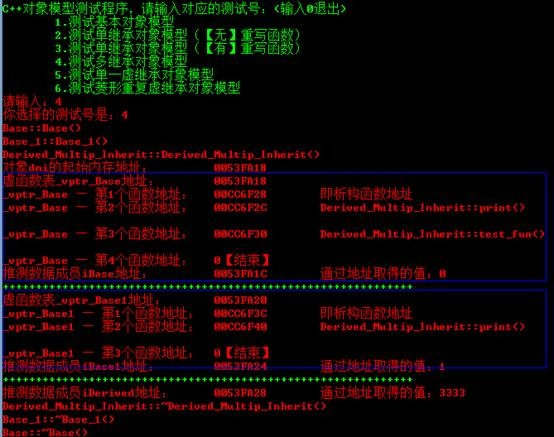# C++对象模型中加入虚继承

## 简单虚继承（无重复继承情况）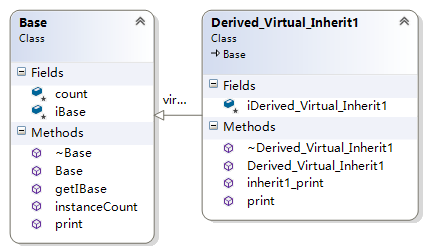Derived_Virtual_Inherit1的对象模型如下图：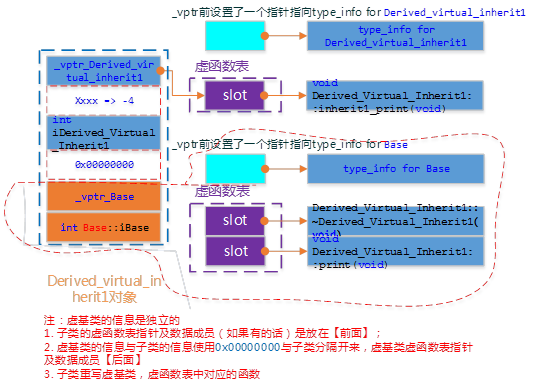测试代码： void test_single_vitrual_inherit(){    Derived_Virtual_Inherit1 dvi1(88888);    cout << "对象dvi1的起始内存地址：\t\t" << &dvi1 << endl;    cout << "虚函数表_vptr_Derived..地址：\t\t" << (int*)(&dvi1) << endl;    cout << "_vptr_Derived — 第1个函数地址：\t" << (int*)*(int*)(&dvi1) << endl;    typedef void(*Fun)(void);    Fun pFun = (Fun)*((int*)*(int*)(&dvi1));    pFun();    cout << endl;    cout << "_vptr_Derived — 第2个函数地址：\t" << *((int*)*(int*)(&dvi1) + 1) << "【结束】\t";    cout << endl;    cout << "=======================：\t" << ((int*)(&dvi1) +1) << "\t通过地址取得的值：" << (int*)*((int*)(&dvi1) +1) << "\t" <<*(int*)*((int*)(&dvi1) +1) << endl;    cout << "推测数据成员iDerived地址：\t" << ((int*)(&dvi1) +2) << "\t通过地址取得的值：" << *((int*)(&dvi1) +2) << endl;    cout << "=======================：\t" << ((int*)(&dvi1) +3) << "\t通过地址取得的值：" << *((int*)(&dvi1) +3) << endl;    cout << "虚函数表_vptr_Base地址：\t" << ((int*)(&dvi1) +4) << endl;    cout << "_vptr_Base — 第1个函数地址：\t" << (int*)*((int*)(&dvi1) +4) << "\t即析构函数地址" << endl;    cout << "_vptr_Base — 第2个函数地址：\t" << ((int*)*((int*)(&dvi1) +4) +1) << "\t";    pFun = (Fun)*((int*)*((int*)(&dvi1) +4) +1);    pFun();    cout << endl;    cout << "_vptr_Base — 第3个函数地址：\t" << ((int*)*((int*)(&dvi1) +4) +2) << "【结束】\t" << *((int*)*((int*)(&dvi1) +4) +2);    cout << endl;    cout << "推测数据成员iBase地址：\t\t" << ((int*)(&dvi1) +5) << "\t通过地址取得的值：" << *((int*)(&dvi1) +5) << endl;}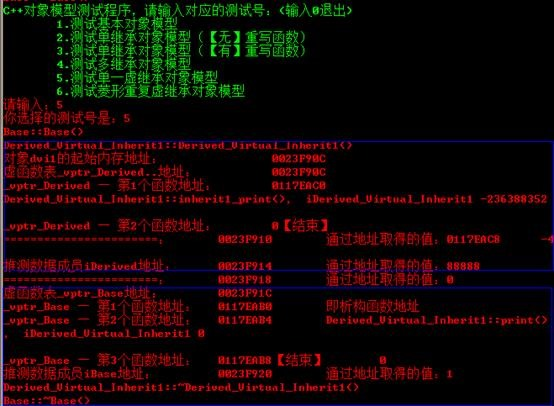## 菱形继承（含重复继承、多继承情况）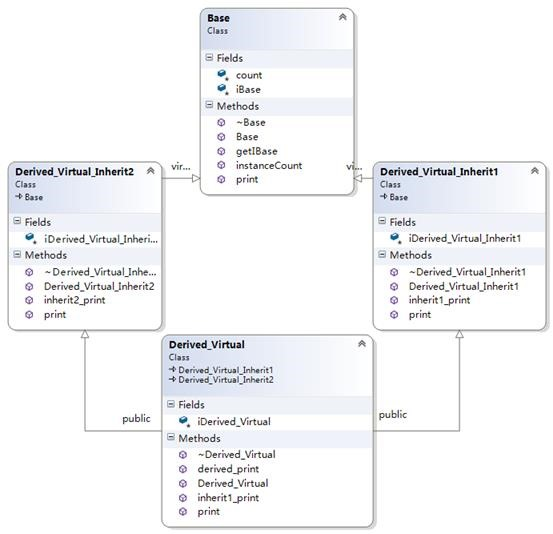Derived_Virtual的对象模型如下图：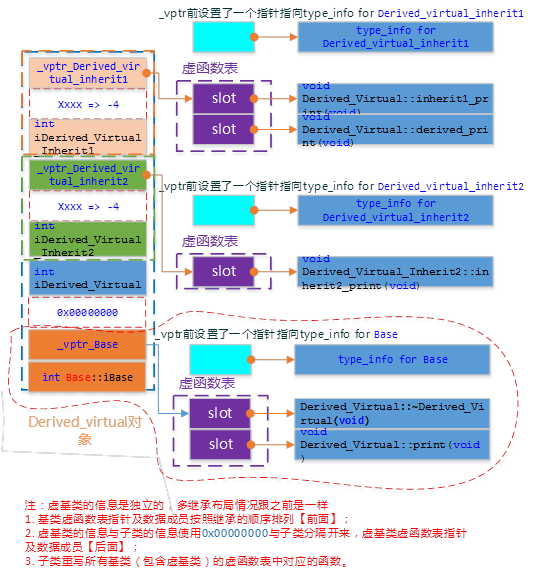测试代码： void test_multip_vitrual_inherit(){    Derived_Virtual dvi1(88888);    cout << "对象dvi1的起始内存地址：\t\t" << &dvi1 << endl;    cout << "虚函数表_vptr_inherit1地址：\t\t" << (int*)(&dvi1) << endl;    cout << "_vptr_inherit1 — 第1个函数地址：\t" << (int*)*(int*)(&dvi1) << endl;    typedef void(*Fun)(void);    Fun pFun = (Fun)*((int*)*(int*)(&dvi1));    pFun();    cout << endl;    cout << "_vptr_inherit1 — 第2个函数地址：\t" << ((int*)*(int*)(&dvi1) + 1) << endl;    pFun = (Fun)*((int*)*(int*)(&dvi1) + 1);    pFun();    cout << endl;    cout << "_vptr_inherit1 — 第3个函数地址：\t" << ((int*)*(int*)(&dvi1) + 2) << "\t通过地址取得的值：" << *((int*)*(int*)(&dvi1) + 2) << "【结束】\t";    cout << endl;    cout << "======指向=============：\t" << ((int*)(&dvi1) +1) << "\t通过地址取得的值：" << (int*)*((int*)(&dvi1) +1)<< "\t" <<*(int*)*((int*)(&dvi1) +1) << endl;    cout << "推测数据成员iInherit1地址：\t" << ((int*)(&dvi1) +2) << "\t通过地址取得的值：" << *((int*)(&dvi1) +2) << endl;    //    cout << "虚函数表_vptr_inherit2地址：\t" << ((int*)(&dvi1) +3) << endl;    cout << "_vptr_inherit2 — 第1个函数地址：\t" << (int*)*((int*)(&dvi1) +3) << endl;    pFun = (Fun)*((int*)*((int*)(&dvi1) +3));    pFun();    cout << endl;    cout << "_vptr_inherit2 — 第2个函数地址：\t" << (int*)*((int*)(&dvi1) +3) + 1 <<"\t通过地址取得的值：" << *((int*)*((int*)(&dvi1) +3) + 1) << "【结束】\t" << endl;    cout << endl;    cout << "======指向=============：\t" << ((int*)(&dvi1) +4) << "\t通过地址取得的值：" << (int*)*((int*)(&dvi1) +4) << "\t" <<*(int*)*((int*)(&dvi1) +4)<< endl;    cout << "推测数据成员iInherit2地址：\t" << ((int*)(&dvi1) +5) << "\t通过地址取得的值：" << *((int*)(&dvi1) +5) << endl;    cout << "推测数据成员iDerived地址：\t" << ((int*)(&dvi1) +6) << "\t通过地址取得的值：" << *((int*)(&dvi1) +6) << endl;    cout << "=======================：\t" << ((int*)(&dvi1) +7) << "\t通过地址取得的值：" << *((int*)(&dvi1) +7) << endl;    //    cout << "虚函数表_vptr_Base地址：\t" << ((int*)(&dvi1) +8) << endl;    cout << "_vptr_Base — 第1个函数地址：\t" << (int*)*((int*)(&dvi1) +8) << "\t即析构函数地址" << endl;    cout << "_vptr_Base — 第2个函数地址：\t" << ((int*)*((int*)(&dvi1) +8) +1) << "\t";    pFun = (Fun)*((int*)*((int*)(&dvi1) +8) +1);    pFun();    cout << endl;    cout << "_vptr_Base — 第3个函数地址：\t" << ((int*)*((int*)(&dvi1) +8) +2) << "【结束】\t" << *((int*)*((int*)(&dvi1) +8) +2);    cout << endl;    cout << "推测数据成员iBase地址：\t\t" << ((int*)(&dvi1) +9) << "\t通过地址取得的值：" << *((int*)(&dvi1) +9) << endl;}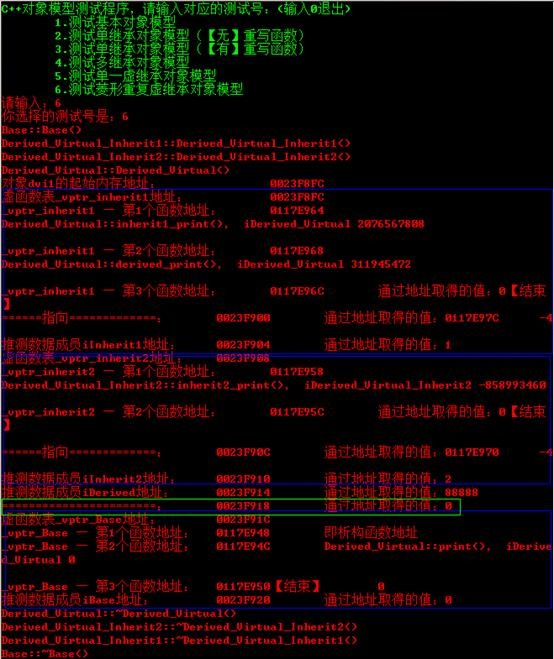# 如何访问成员？

## 对象大小问题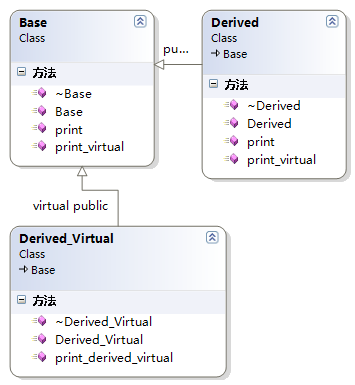l  Derived继承Base

l  Derived_Virtual虚继承Base

 测试对象大小： void test_size(){    Base b;    Derived d;    Derived_Virtual dv;    cout << "sizeof(b):\t" << sizeof(b) << endl;    cout << "sizeof(d):\t" << sizeof(d) << endl;    cout << "sizeof(dv):\t" << sizeof(dv) << endl;}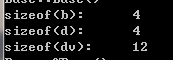空类Empty： #pragma onceclass Empty{public:    Empty(void);    ~Empty(void);};

Empty psizeof(p)的大小是多少？事实上并不是空的，它有一个隐晦的1byte，那是被编译器安插进去的一个char这将使得这个class的两个对象得以在内中有独一无二的地址

## 静态绑定与动态绑定

l  每个对象都将增大，增大量为存储虚函数表指针的大小；

l  对于每个类，编译器都创建一个虚函数地址表；

l  对于每个函数调用，都需要执行一项额外的操作，即到虚函数表中查找地址。

# 多态如何实现?

## 多态的实现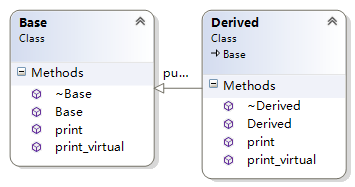void print() const;

virtual void print_virtual() const;

 测试多态代码： void test_polmorphisn(){    Base b;    Derived d;        b = d;    b.print();    b.print_virtual();     Base *p;    p = &d;    p->print();    p->print_virtual();}

l  b.print();b.print_virtual();不能实现多态是因为通过基类对象调用，而非指针或引用所以不能实现多态。

l  p->print();不能实现多态是因为，print函数没有声明为虚函数（virtual，派生类中也定义了print函数只是隐藏了基类的print函数。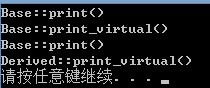## 为什么析构函数设为虚函数是必要的

 测试析构函数： void test_vitual_destructor(){    Base *p = new Derived();    delete p;}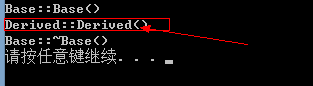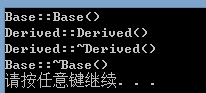# 相关资料

     深度探索C++对象模型，侯捷

     测试代码下载：https://github.com/saylorzhu/CppObjectDataModelTestCode

《深度探索C++对象模型》读书笔记（1）

《深度探索C++对象模型》读书笔记（1）。 在C++中，有两种class data members：static和nonstatic，以及三种class member functions：static、nonstatic和virtual.已知下面这个class Point声...

2012/10/08
84
0
C++对象内存模型---数据封装

“C++是C的超集”，这句话里包含了两个问题： 1. C++兼容C； 2. C++在C之上进行了扩展； 那C++在C之上进行了哪些扩展呢？众所周知，C++内置了众多编程机制、支持众多编程风格：面向过程、基于...

rexlv
2013/05/05
438
0
C语言编程基础新手学习经典练习题和答案详解入门必备

C语言是面向过程的，而C＋＋是面向对象的 C和C++的区别： C是一个结构化语言，它的重点在于算法和数据结构。C程序的设计首要考虑的是如何通过一个过程，对输入（或环境条件）进行运算处理得到...

2018/05/13
0
0
C语言/C++编程基础入门学习重要知识之i++和++i的区别

C语言是面向过程的，而C＋＋是面向对象的 C和C++的区别： C是一个结构化语言，它的重点在于算法和数据结构。C程序的设计首要考虑的是如何通过一个过程，对输入（或环境条件）进行运算处理得到...

2018/03/17
0
0
C#转C++的一点分享

2014/01/17
4K
11

OSChina 周六乱弹 —— 早上儿子问我他是怎么来的

Osc乱弹歌单（2019）请戳（这里） 【今日歌曲】 @凉小生 ：#今日歌曲推荐# 少点戾气，愿你和这个世界温柔以待。中岛美嘉的单曲《僕が死のうと思ったのは (曾经我也想过一了百了)》 《僕が死の...

2.2K
14
Excption与Error包结构，OOM 你遇到过哪些情况，SOF 你遇到过哪些情况

Throwable 是 Java 中所有错误与异常的超类，Throwable 包含两个子类，Error 与 Exception 。用于指示发生了异常情况。 Java 抛出的 Throwable 可以分成三种类型。 被检查异常（checked Exc...

Garphy

38
0

FAT_mt

40
0

61
0
tabel 中含有复选框的列 数据理解

1、el-ui中实现某一列为复选框 实现多选非常简单: 手动添加一个el-table-column，设type属性为selction即可； 2、@selection-change事件：选项发生勾选状态变化时触发该事件 <el-table @sel...

everthing

21
0